true
true

Naresh SawlaniSector 34, Faridabad, India - 121003# Naresh Sawlani## Experienced Teacher

Sector 34, Faridabad, India - 121003.

5.0

UrbanPro Rating

Details verified of Naresh Sawlani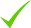IdentityEducation

Know how UrbanPro verifies Tutor details

Identity is verified based on matching the details uploaded by the Tutor with government databases.

## Overview

Dynamic, versatile, hands-on Learning & Development, Project Management, Educator and Training Professional with a passion of imparting and sharing knowledge and experience with future and current management professional and business leaders.
Have trained / mentored over 2000 professionals in various technical and project management disciplines.
Lectured into reputable management institutes as visiting faculty. Impart knowledge and corporate leanings of Marketing, Strategy, HRM and Project Management to the future business professionals.
Credentials hold: PGDBM (Marketing), PMP, B.Com, ITIL, Facet 5.

Sindhi

English

Hindi

## Education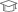Tolani Institute of Management Studies, Gujarat 1999Project Management Institute (PMI) 2008

Project Management Professional (PMP)

Sector 34, Faridabad, India - 121003

## Verified Info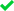ID VerifiedEducation VerifiedPhone VerifiedEmail Verified

Report this Profile

Is this listing inaccurate or duplicate? Any other problem?

Type the letters as shown below *

Please enter the letters as show below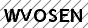Class I-V Tuition

Class LocationStudent's HomeTutor's HomeOnline (video chat via skype, google hangout etc)

Years of Experience in Class I-V Tuition

7

Experience in School or College

Regularly take classes in a private institute in Lajpat Nagar

Teaching Experience in detail in Class I-V Tuition

I love to teach and see the progress my students make. Classes Teach: Grade 4 - Grade 9 (Maths, Science, English) Location: Student's Home (in the vicinity of 5-6 KM of Ashoka Enclave), At Home, Online. When you schedule a trial lesson with me, we’ll go over your goals and make a plan. I am very flexible to your needs. I’ll focus a lesson on exactly what you want to learn. I tend to make the learning process go smoothly. Can start the session the same day on agreeing to the contract. 1st Demo class will be free. Thanks for your time. Please contact if suits your requirement.

## Gallery (1)

Taking a MBA class

## Reviews

this is test message this is test message this is test message this is test message this is test message this is test message this is test message

No Reviews yet! Be the first one to Review

## FAQs

1. Which classes do you teach?

I teach Class 8 Tuition and Class I-V Tuition Classes.

2. Do you provide a demo class?

Yes, I provide a free demo class.

3. How many years of experience do you have?

I have been teaching for 7 years.

## Answers by Naresh Sawlani (2)

Answered on 06/08/2019 CBSE/Class 9/Mathematics/Unit 5-Mensuration/Heron's formula/NCERT Solutions/Exercise 12.2

For finding the area of the paper used, determine the area of each part separately and then find the sum of the areas to get the area of used paper. For region I (Triangle) Length of the sides of the triangle section I = a=5cm, b=1cm and c=5cm Semi Perimeter of the triangle, s =( a+b+c)/2s=(5 + 5 + 1)/2=... ...more

For finding the area of the paper used, determine the area of each part separately and then find the sum of the areas to get the area of used paper.

For region I (Triangle)

Length of the sides of the triangle section I = a=5cm, b=1cm and c=5cm

Semi Perimeter of the triangle,
s =( a+b+c)/2
s=(5 + 5 + 1)/2= 11/2cm

Semi perimeter = 11/2 cm = 5.5cm

Using heron’s formula,
Area of section I = √s (s-a) (s-b) (s-c)

= √5.5(5.5 – 5) (5.5 – 5) (5.5 – 1) cm²

= √5.5 × 0.5 × 0.5 × 4.5 cm²

= √5.5 × 0.5 × 0.5 × 4.5 cm²

= 0.75√11 cm²= 0.75 ×3.32 cm²
= 2.49 cm² (approx)

Section II( rectangle)

Length of the sides of the rectangle of section II = 6.5cm and 1cm

Area of section II = l ×b= 6.5 × 1
= 6.5cm²

Section III is an isosceles trapeziumIn ? AMD
AM + NB = AB – MN = 1cm
Therefore, AM = 0.5cm
MD²= 1² – 0.5²
MD²= 1- 0.25= 0.75
MD = √0.75= √75/100=√3/4cm

Now, area of trapezium = ½(sum of parallel sides)×height

=½×(AB+DC)×MD

=½×(2+1)×√3/4

= ½(3)×√(3/4)= ½×3×√3×2=(3/4)√3

= (3/4)×1.73= 1.30cm²(approx)
[√3=1.73....]

Hence, area of trapezium = 1.30cm²

Section IV and V are 2 congruent right angled triangles with base 6cm and height 1.5cm

Area of region IV and V = 2 (½ × 6 × 1.5)cm² = 9cm²

Total area of the paper used = Area I + Area II + Area III + (Area IV + Area V) = (2.49+ 6.5 + 1.30 + 9)

= 19.3 cm² (approx)

Dislike Bookmark

Answered on 06/08/2019 CBSE/Class 9/Mathematics/Unit 5-Mensuration/Heron's formula/NCERT Solutions/Exercise 12.1

Heron's Formula for the area of a triangle(Hero's Formula) A method for calculating the area of a triangle when you know the lengths of all three sides. Let a=15m,b=11m,c=6m be the lengths of the sides of a triangle. The area is given by: Area = √p(p−a)(p−b)(p−c) where p is half... ...more

Heron's Formula for the area of a triangle(Hero's Formula)

A method for calculating the area of a triangle when you know the lengths of all three sides.

Let a=15m,b=11m,c=6m be the lengths of the sides of a triangle. The area is given by:

Area = √p(p−a)(p−b)(p−c)

where p is half the perimeter, or (a+b+c)/2 = (15+11+6)/2 =16m

Therefore, Area = √16(16-15)(16-11)(16-6) = √16(1)(5)(10) =20√2 = 20 × 1.414 = 28.28 m²

Dislike Bookmark
Class I-V Tuition 5.0

Class LocationStudent's HomeTutor's HomeOnline (video chat via skype, google hangout etc)

Years of Experience in Class I-V Tuition

7

Experience in School or College

Regularly take classes in a private institute in Lajpat Nagar

Teaching Experience in detail in Class I-V Tuition

I love to teach and see the progress my students make. Classes Teach: Grade 4 - Grade 9 (Maths, Science, English) Location: Student's Home (in the vicinity of 5-6 KM of Ashoka Enclave), At Home, Online. When you schedule a trial lesson with me, we’ll go over your goals and make a plan. I am very flexible to your needs. I’ll focus a lesson on exactly what you want to learn. I tend to make the learning process go smoothly. Can start the session the same day on agreeing to the contract. 1st Demo class will be free. Thanks for your time. Please contact if suits your requirement.

Class 8 Tuition 5.0

Class LocationStudent's HomeTutor's HomeOnline (video chat via skype, google hangout etc)

Years of Experience in Class 8 Tuition

7

Experience in School or College

Teaching experience of over 3 years

Teaching Experience in detail in Class 8 Tuition

3 years tuition and teaching experience to students from VI-IX Subjects taught: English, Mathematics, Science

this is test message this is test message this is test message this is test message this is test message this is test message this is test message

No Reviews yet! Be the first one to Review

## Answers by Naresh Sawlani (2)

Answered on 06/08/2019 CBSE/Class 9/Mathematics/Unit 5-Mensuration/Heron's formula/NCERT Solutions/Exercise 12.2

For finding the area of the paper used, determine the area of each part separately and then find the sum of the areas to get the area of used paper. For region I (Triangle) Length of the sides of the triangle section I = a=5cm, b=1cm and c=5cm Semi Perimeter of the triangle, s =( a+b+c)/2s=(5 + 5 + 1)/2=... ...more

For finding the area of the paper used, determine the area of each part separately and then find the sum of the areas to get the area of used paper.

For region I (Triangle)

Length of the sides of the triangle section I = a=5cm, b=1cm and c=5cm

Semi Perimeter of the triangle,
s =( a+b+c)/2
s=(5 + 5 + 1)/2= 11/2cm

Semi perimeter = 11/2 cm = 5.5cm

Using heron’s formula,
Area of section I = √s (s-a) (s-b) (s-c)

= √5.5(5.5 – 5) (5.5 – 5) (5.5 – 1) cm²

= √5.5 × 0.5 × 0.5 × 4.5 cm²

= √5.5 × 0.5 × 0.5 × 4.5 cm²

= 0.75√11 cm²= 0.75 ×3.32 cm²
= 2.49 cm² (approx)

Section II( rectangle)

Length of the sides of the rectangle of section II = 6.5cm and 1cm

Area of section II = l ×b= 6.5 × 1
= 6.5cm²

Section III is an isosceles trapeziumIn ? AMD
AM + NB = AB – MN = 1cm
Therefore, AM = 0.5cm
MD²= 1² – 0.5²
MD²= 1- 0.25= 0.75
MD = √0.75= √75/100=√3/4cm

Now, area of trapezium = ½(sum of parallel sides)×height

=½×(AB+DC)×MD

=½×(2+1)×√3/4

= ½(3)×√(3/4)= ½×3×√3×2=(3/4)√3

= (3/4)×1.73= 1.30cm²(approx)
[√3=1.73....]

Hence, area of trapezium = 1.30cm²

Section IV and V are 2 congruent right angled triangles with base 6cm and height 1.5cm

Area of region IV and V = 2 (½ × 6 × 1.5)cm² = 9cm²

Total area of the paper used = Area I + Area II + Area III + (Area IV + Area V) = (2.49+ 6.5 + 1.30 + 9)

= 19.3 cm² (approx)

Dislike Bookmark

Answered on 06/08/2019 CBSE/Class 9/Mathematics/Unit 5-Mensuration/Heron's formula/NCERT Solutions/Exercise 12.1

Heron's Formula for the area of a triangle(Hero's Formula) A method for calculating the area of a triangle when you know the lengths of all three sides. Let a=15m,b=11m,c=6m be the lengths of the sides of a triangle. The area is given by: Area = √p(p−a)(p−b)(p−c) where p is half... ...more

Heron's Formula for the area of a triangle(Hero's Formula)

A method for calculating the area of a triangle when you know the lengths of all three sides.

Let a=15m,b=11m,c=6m be the lengths of the sides of a triangle. The area is given by:

Area = √p(p−a)(p−b)(p−c)

where p is half the perimeter, or (a+b+c)/2 = (15+11+6)/2 =16m

Therefore, Area = √16(16-15)(16-11)(16-6) = √16(1)(5)(10) =20√2 = 20 × 1.414 = 28.28 m²

Dislike Bookmark

Naresh Sawlani describes himself as Experienced Teacher. He conducts classes in Class 8 Tuition and Class I-V Tuition. Naresh is located in Sector 34, Faridabad. Naresh takes at students Home, Regular Classes- at his Home and Online Classes- via online medium. He has 11 years of teaching experience . Naresh has completed Master of Business Administration (M.B.A.) from Tolani Institute of Management Studies, Gujarat in 1999 and Project Management Professional (PMP) from Project Management Institute (PMI) in 2008. He is well versed in Sindhi, English and Hindi.

•• Want to learn from Naresh Sawlani?

• Contact Now
X

Recommended Profiles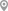Kapur Bawdi, MumbaiMadhurawada, VisakhapatnamNhpc Colony, FaridabadMadipakkam, ChennaiParnasri Palli, KolkataFaridabad NIT, Faridabad

X

Let us shortlist and give the best tutors and institutes.

or

Send Enquiry to Naresh Sawlani

Let Naresh Sawlani know you are interested in their class